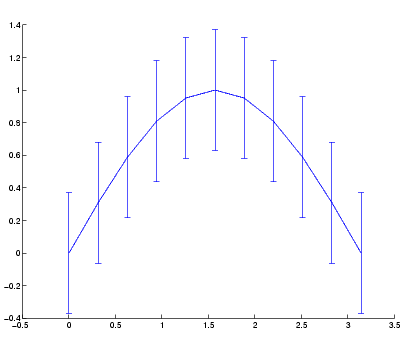MATLAB Function Referenceerrorbar

Plot error bars along a curve

Syntax

• ```errorbar(Y,E)
errorbar(X,Y,E)
errorbar(X,Y,L,U)
errorbar(...,`LineSpec`)
h = errorbar(...)

errorbar('v6',...)
```

Description

Error bars show the confidence level of data or the deviation along a curve.

```errorbar(Y,E) ``` plots `Y` and draws an error bar at each element of `Y`. The error bar is a distance of `E(i)` above and below the curve so that each bar is symmetric and `2*E(i)` long.

```errorbar(X,Y,E) ``` plots `Y` versus `X` with symmetric error bars `2*E(i)` long. `X`, `Y`, `E` must be the same size. When they are vectors, each error bar is a distance of `E(i)` above and below the point defined by `(X(i),Y(i))`. When they are matrices, each error bar is a distance of `E(i,j)` above and below the point defined by `(X(i,j),Y(i,j))`.

```errorbar(X,Y,L,U) ``` plots `X` versus `Y` with error bars `L(i)+U(i)` long specifying the lower and upper error bars. `X`, `Y`, `L`, and `U` must be the same size. When they are vectors, each error bar is a distance of `L(i)` below and `U(i)` above the point defined by `(X(i),Y(i))`. When they are matrices, each error bar is a distance of `L(i,j)` below and `U(i,j)` above the point defined by `(X(i,j),Y(i,j))`.

```errorbar(...,LineSpec) ``` draws the error bars using the line type, marker symbol, and color specified by `LineSpec`.

```h = errorbar(...) ``` returns handles to the errorbarseries objects created. `errorbar` creates one object for vector input arguments and one object per column for matrix input arguments. See errorbarseries properties for more information.

Backward Compatible Version

```hlines = errorbar('v6',...) ``` returns the handles of line objects instead of errorbarseries objects for compatibility with MATLAB 6.5 and earlier.

See Plot Objects and Backward Compatibility for more information.

Remarks

When the arguments are all matrices, `errorbar` draws one line per matrix column. If `X` and `Y` are vectors, they specify one curve.

Examples

Draw symmetric error bars that are two standard deviation units in length.

• ```X = 0:pi/10:pi;
Y = sin(X);
E = std(Y)*ones(size(X));
errorbar(X,Y,E)```

See Also

`LineSpec`, `plot`, `std`

Basic Plots and Graphs for related functions

Error Bounds for related information

See Errorbarseries Properties for property descriptionserror Errorbarseries Properties© 1994-2005 The MathWorks, Inc.